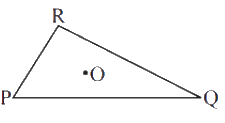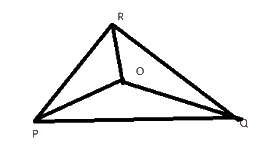# Ex.6.4 Q2 The-Triangle-and-its-Properties Solutions NCERT Maths Class 7

Go back to  'Ex.6.4'

## Question

Take any point $$\rm{}O$$ in the interior of a triangle $$\rm{}PQR$$ Is

(i) $$\rm{}OP + OQ > PQ?$$

(ii) $$\rm{}OQ + OR > QR?$$

(iii) $$\rm{}OR + OP > RP?$$Video Solution
Triangle & Its Properties
Ex 6.4 | Question 2

## Text Solution

What is known?

Triangle $$PQR$$ and point in the interior of triangle.

What is unknown?

(i) $$OP + OQ > PQ?$$

(ii) $$OQ + OR > QR?$$

(iii) $$OR + OP > RP?$$

Reasoning:

This question is straight forward. If $$\rm{}“o”$$ is a point in the interior of a given triangle, then the three triangles can be constructed. You must join all the three vertices of a triangle with the centre $$\rm{}“o”$$. This question is based on a property that in a triangle the sum of lengths of any two sides is always greater than the third side.Steps:

Yes, $$\rm{}POQ$$ forms a triangle

Yes, $$\rm{}OQR$$ forms a triangle.

Yes, $$\rm{}ORP$$ forms a triangle.

Learn from the best math teachers and top your exams

• Live one on one classroom and doubt clearing
• Practice worksheets in and after class for conceptual clarity
• Personalized curriculum to keep up with school